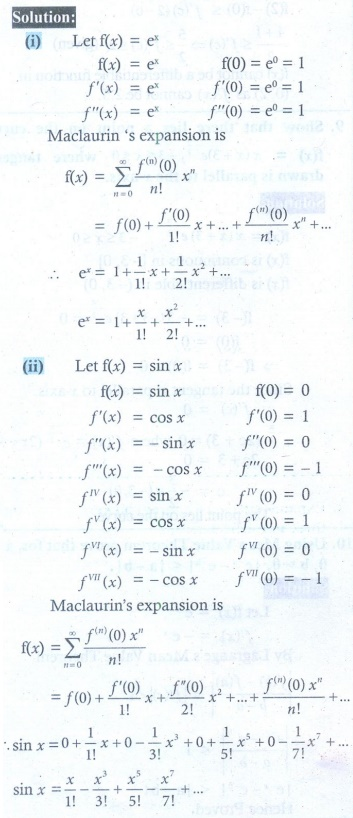Home | | Maths 12th Std | Exercise 7.4 : Series Expansions: MaclaurinŌĆÖs and TaylorŌĆÖs Series

# Exercise 7.4 : Series Expansions: MaclaurinŌĆÖs and TaylorŌĆÖs Series

Maths Book back answers and solution for Exercise questions - Mathematics : Applications of Differential Calculus: Series Expansions: MaclaurinŌĆÖs and TaylorŌĆÖs Series

EXERCISE 7.4

1. Write the Maclaurin series expansion of the following functions:

(i) ex

(ii) sin x

(iii) cos x

(iv) log(1- x) ; ŌĆō1 Ōēż x < 1

(v) tan-1 (x) ; ŌĆō1 Ōēż x Ōēż 1

(vi) cos2 x2. Write down the Taylor series expansion, of the function log x about x = 1 upto three non-zero terms for x > 0.3. Expand sin x in ascending powers x ŌĆō ŽĆ/4 upto three non-zero terms.4. Expand the polynomial f (x) = x2 ŌĆō 3x + 2 in powers of x ŌĆō 1.